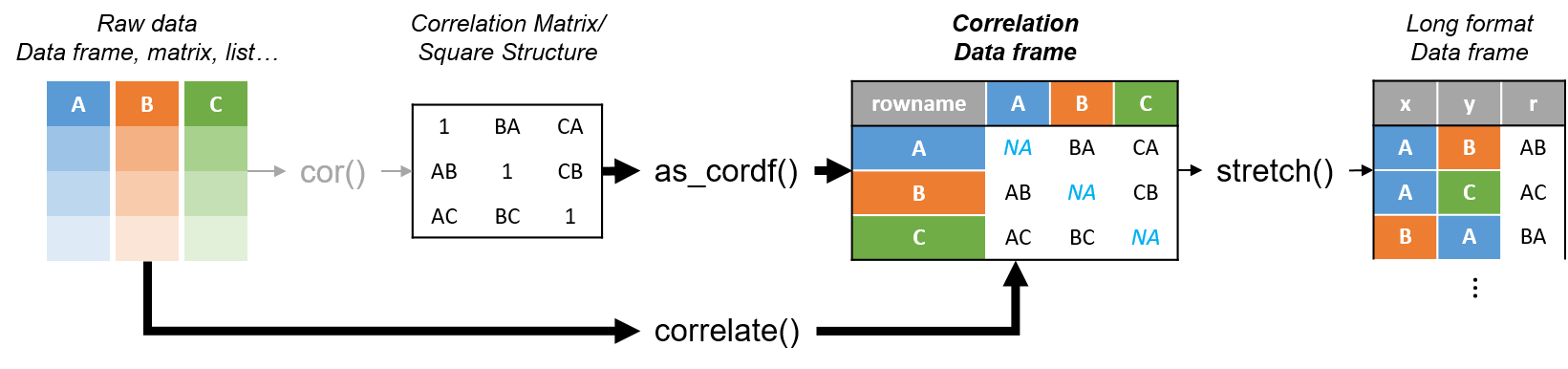R’s standard correlation functionality (`base::cor`) seems very impractical to the new programmer: it returns a matrix and has some pretty shitty defaults it seems. Simon Jackson thought the same so he wrote a `tidyverse`-compatible new package: `corrr`!

Simon wrote some practical R code that has helped me out greatly before (e.g., color palette’s), but this new package is just great. He provides an elaborate walkthrough on his own blog, which I can highly recommend, but I copied some teasers below.Diagram showing how the new functionality of corrr works.

Apart from `corrr::correlate` to retrieve a correlation data frame and `corrr::stretch` to turn that data frame into a long format, the new package includes `corrr::focus`, which can be used to simulteneously select the columns and filter the rows of the variables focused on. For example:

```# install.packages("tidyverse")
library(tidyverse)

# install.packages("corrr")
library(corrr)

# install.packages("here")
library(here)

dir.create(here::here("images")) # create an images directory

mtcars %>%
corrr::correlate() %>%
# use mirror = TRUE to not only select columns but also filter rows
corrr::focus(mpg:hp, mirror = TRUE) %>%
corrr::network_plot(colors = c("red", "green")) %>%
ggplot2::ggsave(
filename = here::here("images", "mtcars_networkplot.png"),
width = 5,
height = 5
)
```With corrr::networkplot you get an immediate sense of the relationships in your data.

Let’s try some different visualizations:

```mtcars %>%
corrr::correlate() %>%
corrr::focus(mpg) %>%
dplyr::mutate(rowname = reorder(rowname, mpg)) %>%
ggplot2::ggplot(ggplot2::aes(rowname, mpg)) +
# color each bar based on the direction of the correlation
ggplot2::geom_col(ggplot2::aes(fill = mpg >= 0)) +
ggplot2::coord_flip() +
ggplot2::ggsave(
filename = here::here("images", "mtcars_mpg-barplot.png"),
width = 5,
height = 5
)
```The tidy correlation data frames can be easily piped into a ggplot2 function call

`corrr` also provides some very helpful functionality display correlations. Take, for instance, `corrr::fashion` and `corrr::shave`:

```mtcars %>%
corrr::correlate() %>%
corrr::focus(mpg:hp, mirror = TRUE) %>%
# converts the upper triangle (default) to missing values
corrr::shave() %>%
# converts a correlation df into clean matrix
corrr::fashion() %>%
```Exporting a nice looking correlation matrix has never been this easy.

Finally, there is the great function of `corrr::rplot` to generate an amazing correlation overview visual in a wingle line. However, here it is combined with `corr::rearrange` to make sure that closely related variables are actually closely located on the axis, and again the upper half is shaved away:

```mtcars %>%
corrr::correlate() %>%
# Re-arrange a correlation data frame
# to group highly correlated variables closer together.
corrr::rearrange(method = "MDS", absolute = FALSE) %>%
corrr::shave() %>%
corrr::rplot(shape = 19, colors = c("red", "green")) %>%
ggplot2::ggsave(
filename = here::here("images", "mtcars_correlationplot.png"),
width = 5,
height = 5
)
```Generate fantastic single-line correlation overviews with `corrr::rplot`

For some more functionalities, please visit Simon’s blog and/or the associated GitHub page. If you copy the code above and play around with it, be sure to work in an Rproject else the here::here() functions might misbehave.